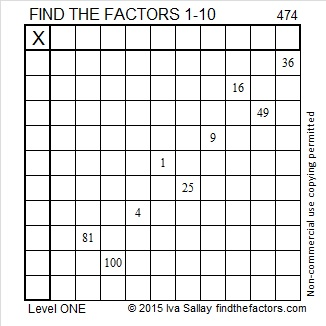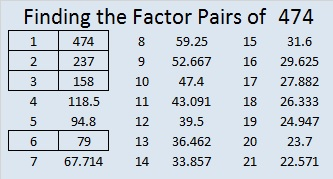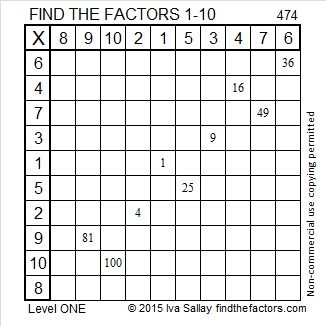# 474 and Level 1

474 is the sum of some consecutive prime numbers which one of my readers listed in the comments. Try to figure out what they are yourself before checking there to see if you were right.Print the puzzles or type the solution on this excel file: 10 Factors 2015-04-27

—————————————————————————————————

• 474 is a composite number.
• Prime factorization: 474 = 2 x 3 x 79
• The exponents in the prime factorization are 1, 1, and 1. Adding one to each and multiplying we get (1 + 1)(1 + 1)(1 + 1) = 2 x 2 x 2 = 8. Therefore 474 has exactly 8 factors.
• Factors of 474: 1, 2, 3, 6, 79, 158, 237, 474
• Factor pairs: 474 = 1 x 474, 2 x 237, 3 x 158, or 6 x 79
• 474 has no square factors that allow its square root to be simplified. √474 ≈ 21.77154—————————————————————————————————